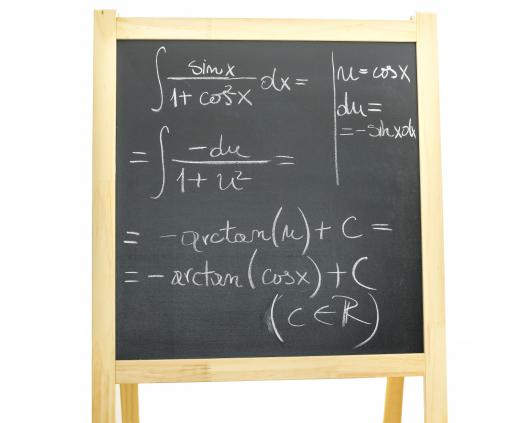Miscellaneous
Fact Checked

# What Are Analytical Methods?

James Doehring
James Doehring

Analytical methods use mathematical principles to fully predict the implications of a theory. They can be used to solve an equation in its entirety without any degree of estimation. They stand in contrast with numerical methods, which can only attain an approximate prediction. Analytical methods are the preferred way to determine the outcome of a hypothesis when associated equations are simple and a precise answer is desired. Numerical methods are used when equations are too complex to solve fully. Previous learnings and skills in applied mathematics and statistics can help make things easier. The more a person is comfortable with numbers, the more they will find analytical methods fun and exciting.

Mathematicians employ analytical methods when they use the basic principles of algebra to solve an equation. If the equation is sufficiently simple, a full solution can be obtained by manipulating the equation in symbolic form. In this case, there is no room for approximation or guesswork—the principles of mathematics always govern which operations can be performed. If the variable in question can be successfully isolated, analytics are the tools that make this possible.

In the equation y = 2x, one can solve for x using analytical methods. To isolate the variable x, both sides of the equation must be divided by the number 2. For any value of y, x can be fully determined with relative ease.Mathematical principles are used to test hypotheses when using analytical methods.

In a simple real-world application of this equation, one might hypothesize that the length of a human foot was twice its width: length = 2*width. This equation implies, necessarily, that width = ½*length. The practical application of the equation may not constitute an accurate theory, but the manipulation of the equation is done using analytical methods. That is, the equation can predict the width of the foot without introducing any approximations.

There are some equations that nobody knows how to solve analytically. For example, many differential equations have no known solutions. A differential equation relates a quantity’s rate of change to its value. Unlike an algebraic equation, differential equations must be solved using calculus. Often times, their results can only be approximated.

Numerical methods are used to solve a number of practical problems. Many businesses try to optimize their sales using numerical methods to approximate market conditions. They can try to predict the outcome of various different business strategies, but they can’t generally use analytics. Making analytical predictions, like in the case of the human foot dimensions, would require one or more equations that related different variables. The market is generally very complicated and has too many variables to be modeled in this way. Although difficult, these methods can be learned with enough time and a highly qualified mentor. With the right motivations and an exceptional online tutor, any student can learn something as complex as differential or algebraic equations.

## You might also Like